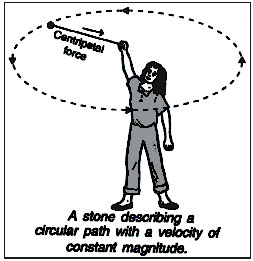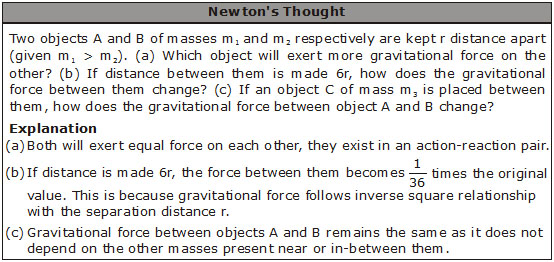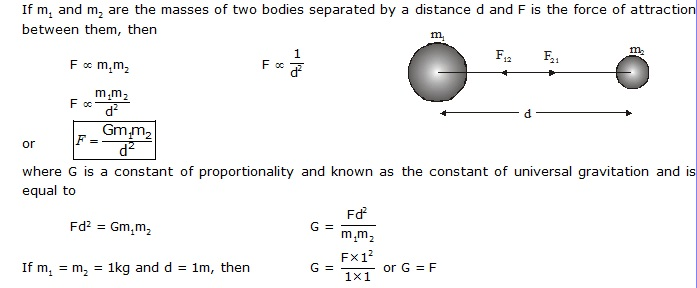## NCERT 9TH CLASS SCIENCE CHAPTER GRAVITATION

Orbit : The paths followed by the planets around the sun and by the satellites around the planets are known as orbits.
According to Newton's first law of motion, these planets and satellites can move in circular orbits only if some force is acting on them. The mystery of the motion of all these heavenly bodies (i.e. planets         and satellites) in nearly circular orbits was solved by Isaac Newton when he observed that an apple fall from a tree towards the earth. Therefore, he proposed that all particles or objects in the universe               attract each other in the same manner as the earth attracted the apple.
"The force of attraction between any two particles in the universe is called gravitation or gravitation force".

Centripetal force :- The force act towards the centre is called centripetal force.Example: Consider a girl whirling a stone along a circular
path. If the girl releases the stone at some point, the stone flies off along the tangent,

at that point on the circular path. Let us discuss this observation carefully.
Before the release of thread, the stone was moving with a certain uniform speed and

changed its direction at every point. Because of the change in direction, it moved with a

variable velocity and has some definite acceleration. The force that causes this acceleration and

makes the stone move along the circular path, acts towards the centre, i.e., towards the hand of

the girl. This force is called centripetal force. When the thread is released, the stone
does not experience the centripetal force and flies off along a straight line. This straight line

is always tangent to the circular path.### NEWTON'S UNIVERSAL LAW OF GRAVITATION

Every particle in the universe attracts every other particle with of force which is directly proportional to the product of two masses and inversely proportional to the square of the distance between them. The     direction of the force is along the line joining the two masses.i.e. Universal gravitational constant is the force of attraction (in newton) between two bodies of mass 1(kg) each lying 1(m) distance apart.

CHARACTERISTICS OF GRAVITATION FO